SEARCH HOMEMath Central Quandaries & QueriesQuestion from Jana, a student: Hello, my name is Jana, i have asked a question here previously about how to count in base-5. I am happy now because i can convert any number now and it is very easy for me. The only thing i am having trouble with is understanding how to do a maths sum or times base-5 numbers. it is just a bit hard for me. So do you think you could explain how to do a sum in base-5 and how to do a multiplication sum. Please help...Thank you Jana!!Hi Jana,

I'm glad you found Stephen's note on counting in different bases helpful.

One thing you need to keep in mind when working in base five is that the only digits you have are 0, 1, 2, 3 and 4. The other digits we work with, like 6 and 9 are just meaningless squiggles when working in base five. In what I am going to do I will only write digits 0, 1, 2, 3 and 4 except in one place. I will write a subscript of 5 on some numbers to remind us that we are working in base five but the subscript 5 is not part of the number. So let's add 1325 and 4415.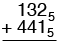Add the units column. 2 + 1 = 3.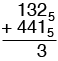Now add the second column. 3 plus 4 is seven. (Notice that I don't write the meaningless squiggle 7.) Seven is 125 so , just as in adding in base 10, I write down the 2 and carry the 1.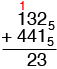Finally add the third column. 4 plus 1 is five plus the carry of 1 gives six. Six is 115 so I get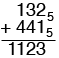Hence 1325 + 4415 = 11235

Now let's try three base five numbers.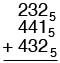The sum of the units column is five. Five is 105 so write down the 0 and carry the 1.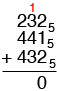The sum of the second column, including the carry is eleven. Eleven is 215 so write down the 1 and carry the 2.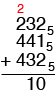Finally, the sum of the last column, including the carry is twelve. Twelve is 225 and hence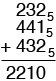Thus 2325 + 4415 + 4325 = 22105

Now try a multiplication yourself. Write down a base 5 multiplication problem just as you would if it were in base 10. Proceed as if you were working in base 10 but remember to convert all the numbers to base 5 before you write them down.

Write back if you need further help,
HarleyMath Central is supported by the University of Regina and The Pacific Institute for the Mathematical Sciences.